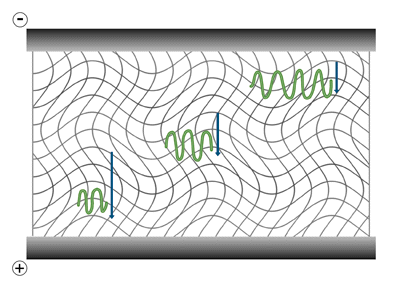# The Mechanical and Electrical Dynamics of Gel Electrophoresis – Intro and Sample Mobility

The term "electrophoresis" refers simply to the movement of particles by an electric force. The first electrophoresis experiments were carried out on molecules in a conductive buffer solution, where the only force acting on the sample was the electric field. The vast majority of current laboratory techniques are performed in a matrix perfused by an aqueous solution of buffer salts. The addition of a matrix (or gel) stabilizes the system against solution turbulence, and extends the range of geometries available to the user. The primary purpose of the matrix, however, is to introduce a sieving action which allows separations based on molecular size. Our discussion will focus on this type of electrophoresis design.

### Sample Mobility

Within an electrophoresis gel, a protein or nucleic acid molecule experiences electric force proportional to its effective charge, Q, and the electric field strength, E. The biomolecule will move with a constant velocity because the electric force on the molecule is met by an opposite force due to the frictional resistance, ƒ, of the matrix.

If electrophoresis occurred in free solution, rather than within a gel, the force of frictional resistance, ƒ, upon biomolecules would follow Stokes' law:

f=6πrvn

where r is the radius of the particle moving with velocity v through a medium of viscosity n. The electromotive force on a molecule, proportional to its charge, is thus opposed by the frictional force ƒ, proportional to its mass. Mobility in free solution would then be the same for molecules of the same charge to mass ratio. Stokes' law, however, is not sufficient to describe the frictional force within a gel matrix. In addition to the viscosity of the medium, also determining frictional resistance are the density and effective "pore size" of the matrix. The result of this combination of factors is that among molecules of the same charge to mass ratio, larger molecules move more slowly in gel electrophoresis and electrophoretic separation occurs by size. In techniques such as denaturing DNA or RNA electrophoresis or SDS-PAGE protein electrophoresis, conditions are maintained so that the charge to mass ratio is virtually equal among sample molecules and separation occurs in a predictable manner based on the size of the molecules.The gel electrophoresis of samples having identical charge to mass ratios results in fractionation by size. Although experiencing greater electric force because of their greater charge, the larger molecules reach force equilibrium with the opposing frictional resistance at a slower speed, thus their lower electrophoretic mobility.

Two additional factors determining sample mobility are the ionic strength of the surrounding solution and the temperature of the gel. To maintain physiological pH and control the net charge on biological molecules in electrophoresis, the solution perfusing the gel matrix contains buffer ions. The ionic strength of this buffer will have a strong effect on the migration of sample molecules. Higher ionic strength in the solution reduces the effective charge of the sample molecules. Therefore, low ionic strength solutions generally correspond to high rates of migration. Electrophoresis in higher ionic strength solutions generally gives slower rates of migration but produces sharper bands. A problem occurs, however, with high ionic strength buffers, when excessive power generation causes the gel temperature to increase. This temperature increase leads to a decrease in the viscosity of the medium, which then leads to increased current and further heating. Buffer evaporation, sample denaturation and convective mixing are typical problems which can result in this scenario.